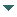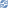# gvSIG

##### Personal tools
You are here: Home Module geom reference
gvSIG DesktopgvSIG Desktop

Cached time 11/21/13 11:24:39# Module geom reference

Up one level
 ◄ Previous Module gvsig reference Next: Related articles ►

geom module contains utilities to manage geometries

Note

### Commons geometries methods

Interface Geometry: This interface is equivalent to the GM_Object specified in ISO 19107. A geometric object shall be a combination of a coordinate geometry and a coordinate reference system.

• area(): Return geometry area
• buffer(distance): Computes a buffer area around this geometry having the given width
• centroid(): Return geometry centroid
• distance(geometry): Returns the minimum distance between this Geometry and the specified geometry.
• getEnvelope(): Returns the minimum bounding box for this Geometry.
• getGeometryType(): Instance of the GeometryType associated to this geometry
• getDimension(): Returns the largest number n such that each direct position in a geometric set can be associated with a subset that has the direct position in its interior and is similar (isomorphic) to Rn, Euclidean n-space

### Geometry types

#### Point

This interface is equivalent to the GM_Point specified in ISO 19107. It is one of the basic data type for a geometric object consisting of one and only one point. They do not have volume, area, length, or any other higher-dimensional analogue.

• getX(): Returns the X coordinate
• getY(): Returns the Y coordinate
• getCoordinateAt(dimension): Gets the coordinate in a concrete dimension
• param dimension: Dimension coordinate to get
• type dimension: integer
• allowed values: 0=X, 1=Y, 2=Z
• setX(value): Sets the X coordinate
• param value: Value of the dimension X
• type value: double
• setY(value): Sets the Y coordinate
• param value: Value of the dimension Y
• type value: double
• setCoordinateAt(dimension, value): Sets a ordinate in a concrete dimension
• param dimension: Dimension coordinate to set
• type dimension: integer
• param value: Value of the dimension
• type value: double

#### Line

This interface is equivalent to the GM_OrientableCurve specified in ISO 19107. Curves are continuous, connected, and have a measurable length in terms of the coordinate system.

• param point: Point to addLayer
• type: Point
• getNumVertices(): Gets the number of vertex of the curve
• getVertex(index): Gets a vertex
• param index: Geometry vertex index
• type index: integer

#### Polygon

This interface is equivalent to the GM_Surface specified in ISO 19107. This is the basis for 2-dimensional geometry

• param point: Point to addLayer
• type: Point
• getNumVertices(): Gets the number of vertex of the curve
• getVertex(index): Gets a vertex
• param index: Geometry vertex index
• type index: integer
• getSurfaceAppearance(): Gets surface appearance
• setSurfaceAppearance(appearance): Sets surface appearance

#### Multigeometry

This interface is equivalent to the GM_Aggregate specified in ISO 19107

• getPrimitiveAt(int i): Returns one of the Primitive's that is in a concrete position. - param i: geometry position - type i: integer
• getPrimitivesNumber(): Returns the number of Primitive's that composes this multi geometry.

#### Geometry util functions

• createGeometry(type[, subtype]): Create a new geometry with a concrete type and subtype
• param type: geometry type
• type type: integer
• param subtype: (Optional) geometry subtype
• type type: integer
• return: geometry
• rtype: Geometry
• createPoint([x][, y][, subtype]): Create a new point with a subtype dimensions and sets the value for 'X' and 'Y' coordinates. If is used without parameters will return new Point2D with (0,0) coordinates
• param x:(Optional) X coordinate value
• type x: double
• param y:(Optional) Y coordinate value
• type y: double
• param subtype: (Optional) geometry dimensions
• type: integer
• return: Point
• rtype: Point
• createMultiPoint(points[, subtype]): Create a new multipoint with a subtype from a list values of X and Y like ([x1, y1], [x2, y2], ...., [xn, yn])
• param points: list of tuples with X and Y values
• type points: list
• param subtype: (Optional) geometry dimensions
• type: integer
• return: multipoint
• rtype: multipoint
 ◄ Previous Module gvsig reference Next: Related articles ►